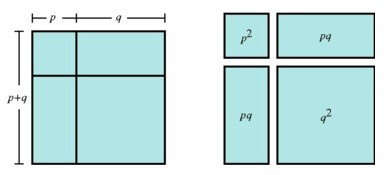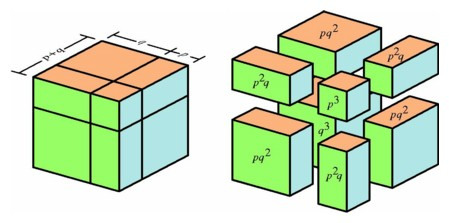The Geometry of the Binomial Theorem

The binomial theorem gives a famous algebraic formula for the sum of two numbers raised to a power. There is a corresponding geometric expression for the volume of an n-dimensional cube with each edge broken into two segments. Earlier in this chapter we considered squares having side length m and area m2. If we express m as a sum of two numbers p and q, then the algebraic operation of writing m as a sum p + q corresponds to the geometric operation of breaking a segment of length m into two segments of length p and q. If we break a vertical edge of a square having side length m into two segments and draw a horizontal line through the break point, we decompose the square into two rectangles. Then doing the same with a horizontal edge produces a decomposition of the square into four pieces: a square of side length p, a second square of side length q, and two rectangles having side lengths p and q. The four parts of this geometric decomposition correspond to the four terms of the expansion of the square of a binomial. The algebraic problem of finding the square of expression p + q is equivalent to the geometric problem of finding the area of a square having side length p + q. The rules of algebra enable us to multiply out the square of a binomial, without having to appeal to a geometric diagram:

 (p + q)2 = (p + q)(p + q) = p(p + q) + q(p + q) = p2 + pq + qp + q2

We may combine the two terms pq and qp to obtain the familiar expression for the square of a binomial:

(p + q)2 = p2 + 2pq + q2

A glance at the diagram below makes the relationship very clear. Each term of the expression p2 + pq + qp + q2 gives the area of one of the four pieces that form the square. The areas of the pieces add up to the area of the original square, namely (p + q)2.A geometric interpretation of the square of a binomial.

There is a similar pattern relating the volume of a cube having side length p + q in three-space to the third power of the binomial p + q. The algebraic procedure is direct enough:

 (p + q)3 = (p + q)(p + q)2 = p(p + q)2 + q(p + q)2 = p(p2 + 2pq + q2) + q(p2 + 2pq + q2) = (p3 + 2p2q + pq2) + (qp2 + 2pq2 + q3) = p3 + 3p2q + 3pq2 + q3

Once again, this argument can take place independent of a geometric interpretation, but nonetheless it is useful to give one. A cube having side length p + q can be decomposed into eight pieces: a cube of side length p, another of side length q, and six square prisms, three having height p and square base of side length q, and three having height q and square base of side length p.A geometric interpretation of the cube of a binomial.

What happens to these algebraic and geometric patterns in the fourth dimension? As above, we can obtain the algebraic expression

(p + q)4 = p4 + 4p3q + 6p2q2 + 4pq3 + q4

which does not rely on any geometric argument. Nevertheless we can consider a hypercube of side length p + q and describe a geometric decomposition analogous to the three-dimensional case. This decomposition will have 16 pieces: a hypercube of side length p, another of side length q, and 8 four-dimensional prisms with cubical bases, 4 having height p and cubical base of side length q, and 4 having height q and cubical base of side length p. In addition there are 6 new objects, four-dimensional prisms having at each vertex two edges of length p and two of length q. Thus the geometric pattern generalizes even though we cannot construct in our space a four-dimensional model that will illustrate the decomposition.

The sequences of coefficients that appear in the expansion of binomials are the rows in Pascal's triangle, the famous number pattern that arises in the theory of combinations. Each number in the pattern is the sum of the two numbers above it. We will see these same patterns of numbers occurring in several different guises as we analyze the structures of objects in higher dimensions.

 1 1 1 1 2 1 1 3 3 1 1 4 6 4 1